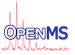OpenMS
GaussFitter Class Reference

Implements a fitter for Gaussian functions. More...

#include <OpenMS/MATH/STATISTICS/GaussFitter.h>

Collaboration diagram for GaussFitter:
[legend]

## Classes

struct  GaussFitResult
struct of parameters of a Gaussian distribution More...

## Public Member Functions

GaussFitter ()
Constructor. More...

virtual ~GaussFitter ()
Destructor. More...

void setInitialParameters (const GaussFitResult &result)
sets the initial parameters used by the fit method as initial guess for the Gaussian More...

GaussFitResult fit (std::vector< DPosition< 2 > > &points) const
Fits a Gaussian distribution to the given data points. More...

## Static Public Member Functions

static std::vector< double > eval (const std::vector< double > &evaluation_points, const GaussFitResult &model)
Evaluate the current Gaussian model at the specified points. More...

## Protected Attributes

GaussFitResult init_param_

## Private Member Functions

GaussFitter (const GaussFitter &rhs)
Copy constructor (not implemented) More...

GaussFitteroperator= (const GaussFitter &rhs)
Assignment operator (not implemented) More...

## Detailed Description

Implements a fitter for Gaussian functions.

This class fits a Gaussian distribution to a number of data points. The results as well as the initial guess are specified using the struct GaussFitResult.

The complete Gaussian formula with the fitted parameters can be transformed into a gnuplot formula using getGnuplotFormula after fitting.

## ◆ GaussFitter() [1/2]

 GaussFitter ( )

Constructor.

## ◆ ~GaussFitter()

 virtual ~GaussFitter ( )
virtual

Destructor.

## ◆ GaussFitter() [2/2]

 GaussFitter ( const GaussFitter & rhs )
private

Copy constructor (not implemented)

## ◆ eval()

 static std::vector eval ( const std::vector< double > & evaluation_points, const GaussFitResult & model )
static

Evaluate the current Gaussian model at the specified points.

Returns the intensities (i.e. probabilities scaled by the factor 'A') of the PDF at the given positions. This function can be called with any set of parameters, e.g. the initial parameters (to get a 'before-fit' status), or after fitting.

## ◆ fit()

 GaussFitResult fit ( std::vector< DPosition< 2 > > & points ) const

Fits a Gaussian distribution to the given data points.

Parameters
 points the data points used for the Gaussian fitting
Exceptions
 Exception::UnableToFit is thrown if fitting cannot be performed

## ◆ operator=()

 GaussFitter& operator= ( const GaussFitter & rhs )
private

Assignment operator (not implemented)

## ◆ setInitialParameters()

 void setInitialParameters ( const GaussFitResult & result )

sets the initial parameters used by the fit method as initial guess for the Gaussian

## ◆ init_param_

 GaussFitResult init_param_
protected